Courses

# Operation Of Three Phase Transformers Electrical Engineering (EE) Notes | EduRev

## Electrical Engineering (EE) : Operation Of Three Phase Transformers Electrical Engineering (EE) Notes | EduRev

The document Operation Of Three Phase Transformers Electrical Engineering (EE) Notes | EduRev is a part of the Electrical Engineering (EE) Course Electrical Machines.
All you need of Electrical Engineering (EE) at this link: Electrical Engineering (EE)

Operation of Three Phase Transformers
(Refer Slide Time: 01:13)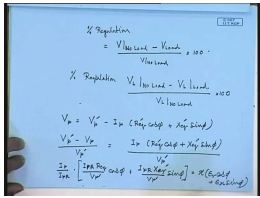In the last lecture you have seen how to find out the terminal voltage of the 3 phase transformer, when it is loaded, it is similar to what we have done in a single phase transformer. In fact, in a single phase transformer we have defined the quantity percentage regulation of a transformer as the terminal voltage at loaded condition. V at No load minus V at Load divided by V at No load into 100.

A similar definition of percentage regulation applies for three phase transformer; however, in this case percentage regulation is defined as line voltage at No load minus line voltage at load divided by line voltage at No load into 100; however, the formula will be the same as we have seen in our last lecture the loaded terminal voltage was given by V p equal to this following that V p the loaded phase voltage V p equal to no load phase voltage V p dash, which is the applied voltage minus I p R e q cos phi plus X e q dash sin phi.

Hence V p dash minus V p by V p dash equal to I p into r e q cos phi plus x e q dash sin phi by V p dash, which can be written as I p by I p rated into I p rated R e q dash by V p dash cos phi plus I p rated X e q dash V p dash sin phi, which will be equal to x in to epsilon r cos phi plus epsilon x sin phi. Where epsilon r is the per unit series resistance of the 3 phase transformer epsilon x is the per unit series resistance of the 3 phase transformer and x is the percentage loading.

So, the formula is identical to that of a single phase transformer, the other important operation parameter of a 3 phase transformer as in the case of single phase transformer is its efficiency, and efficiency of a 3 phase transformer can also be found from the no load test data. For example, if we go back to our previous example of that 11 k V by 400 volt 150 k V a 3 phase transformer with this data no load and short circuit test data. we can find out it efficiency under any loading condition.

(Refer Slide Time: 05:46)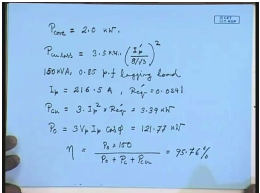So, the no load test had a total power loss of 2 kilowatt. So, the core loss p core of this transformer equal to 2.0 kilowatt. From the short circuit test data we find that the 3 phase power loss at rated line current at 8 ampere line current is 3.5 kilowatt therefore, at any copper loss at any operating condition is equal to 3.5 kilowatt into the load current I p divided by 8 divided by root 3 whole square.

For the particular operating condition that we have said that is 150 k VA which is the full load current full load and 0.85 p f lagging load. You have found that in that case the I p on the low voltage side was two 216.5 ampere, and R e q dash for this transformer was 0.0241. Therefore, p copper loss under the stated condition was 3 into I e square into R e q dash and this comes out to be 3.39 kilowatt.

So, the core loss of the transformer is two kilowatt the copper loss is 3. 39 kilowatt and power output of the transformer p 0 is equal to 3 V p I p cos phi this comes to 121.77 kilowatt. Therefore, the efficiency of the transformer under this operating condition of 150 k VA point 85 p f lagging nu equal to p 0 into 100 divided by p 0 plus p c plus p c u this comes to 95.76 percent. So, we can find also the efficiency of a 3 phase transformer in a manner similar to what we have done for single phase transformers, while these are important performance parameter of a transformer.

It is also extremely important to know what will be the temperature raise of a transformer, when it supplies some load now this cannot be found experimentally from either no load test or short circuit test. Since during no loss there is no current flowing in one of the windings the transformer current is very less, hence the copper loss is almost absent and during short circuit test only a very small fraction of the line voltage is applied therefore, the core loss is almost 0, in order to find the temperature rise of the transformer under certain loading condition. It is necessary to emulate, both the core loss and the copper loss simultaneously. We have seen for single phase transformer, this can be done by the back to back or some test for the hit run test can also be conducted for a 3 phase transformer.

(Refer Slide Time: 10:41)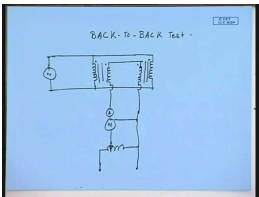So, in a single phase transformer what did we do, for BACK to BACK Test. We supplied the primaries of the transformer from the same supply connected them in parallel, and connected the secondary in series. So, that the induced voltage in the secondary’s oppose each other. This, we connected to the rated supply and this emitter wattmeter connected to a auxiliary source. A similar arrangement has to be done for a 3 phase transformer. So, let us say we have a 3 phase transformer, if any particular site is star connected ,what we should do, we have to connect them those star windings in series ,while the other side has to be connected in parallel.

(Refer Slide Time: 12:20)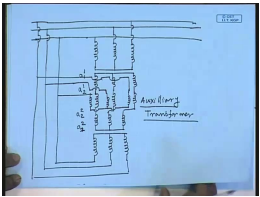So, the following figure shows an arrangement let us say this is the 3 phase main supply line and you have connected the 1 of the winding in parallel this is 1 winding of 1 transformer phase V or L v. This is the other winding of the same transformer. So, the other winding the secondary winding are also star connected. So, these windings are to be connected in series, the polarities should be such that the induced voltages oppose to each other. So, if this is a 1 terminal of transformer 1 and this is a 2 terminal of transformer 1, then this should be a 2 terminal of transformer 2 and this should be a 1 terminal of transformer 2.

So, if you connect them in series the voltages will oppose each other; however, how do we apply the auxiliary voltage phase of that, here we will need a separate auxiliary three phase transformer necessary. So, in order to supply that we will supply from a auxiliary transformer like this. It will not be varaic anymore, it has to be supplied from a separate three winding auxiliary transformer now. If 1 of the windings are delta connected then the arrangement becomes somewhat simpler.

(Refer Slide Time: 16:20)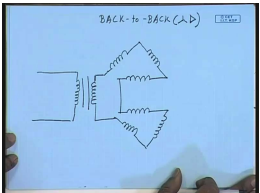Let us say this is the delta connected winding. You open the delta at both the windings at 1 point, and connect these 2 open ends in series. And then you can connect a single phase auxiliary transformer like this, to circulate the necessary current through that. So, this is for back to back test, which star connected transformer this is back to back test with star delta transformer. Now, how do you determine, which connection to use in a particular application, normally star and delta connection at the most common type of the connection. You can see that, if you have a star connected side with its neutral.

(Refer Slide Time: 18:17)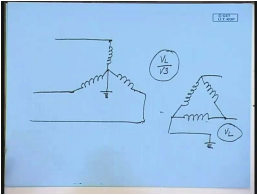If you have a star connected set of winding with this neutral earth, then the maximum voltage that the term windings will have with respect to ground will be V line by root 3. And other hand, if you have a delta connected winding n in the case of a line to ground fault the potential of the other line terminal can go to as high as V l.

Therefore, for the same voltage rating of the winding a star connected winding will require lower insulation, and hence will be somewhat cheaper that is very high voltage transformer generally star connected high voltage side is usually star connected. Now if you have to supply loads which are unbalanced, then it is better to have a star connected side with the neutral made available.

(Refer Slide Time: 20:13)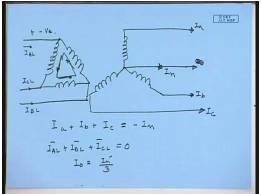So, that the line current can probe through the neutral, if there is a currents are I a, I b and I c. when the neutral current is I n , then I a plus I b plus I c equal to minus I n. Now, if the primary of this is delta connected, then the reflected winding currents I a dash, I b dash and I c dash will also be unbalanced, they will not add up to 0. On the other hand, on the line only positive and negative sequence component can flow, that is the line currents I A L, I C L, I B L must adopt to the 0, this is always 0; however, the induced winding currents are not adopt to 0. So, there is this 0 sequence current I 0 equal to I n dash by 3, that circulates through the close delta. This is necessary to prevent distortion of the secondary side voltage. So, if you have a star connected winding supplying unbalanced load through a neutral connection, and then the other winding should preferably be delta connected.

So, another reason that decides that winding should be star connected or delta connected is the configuration of harmonics, while discussing the magnetisation phenomenon of single phase transformer. We have seen that in a practical transformer the core is operated near the saturation point. Therefore, if the applied voltage is sine wave the flux is also be sine wave; however, the current will no longer be a sine wave and it will have magnetisation current, we will have a predominant hard harmonic component.

(Refer Slide Time: 24:00)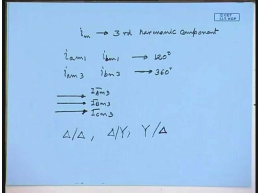Now, the third harmonic the phase angle between I a m 1 and let us say, I b m1 this is 120 degree, but for the third harmonic this is 360 degree. Therefore, the third harmonic magnetisation currents are cophasor therefore, they do not add up to 0. It is something like that 0 sequence component since they do not add up to 0 it is necessary that 1 of the windings provide a path for this third harmonic component to flow. So, that the flux and the induced voltages are almost sinusoidal.

So, the preferred winding connections will be delta delta, delta star or star delta , which we have seen if one side is very high voltage that side should preferably be star connected, the other side may be delta connected. So, when the transmission level the high voltage side winding will usually be the star connected winding, while the other side will be delta connected.

The delta delta winding is used specifically for low voltage transformer that needs to be supply a very large current, because in delta delta winding the winding current is less than the line current therefore, the cross section of the winding becomes smaller. For the same reason the star star connected transformer is not very much in use particularly the isolated neutral star star connected transformer. Let us see, the problem that may occur due to a isolated neutral star star transformer, where the path for this third harmonic current do not exist.

(Refer Slide Time: 27:03)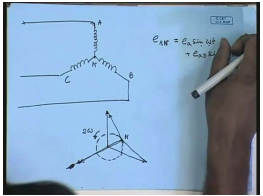So, suppose we have applied a balance three phase voltage, to this isolated neutral winding, the other winding also is star connected and does not provide a return path for the third harmonic current. In which case there will be no third harmonic current, the no load current will be almost sinusoidal and the flux will be non sinusoidal, and the induced voltage e AN will also be non sinusoidal with a predominant third harmonic component. So, e AN can be written as e a sin omega t plus e a 3 sin 3 omega t.

Now, neglecting the impedance of the transformer at no load this should be equal to the applied voltage. So, for all the 3 phases we can draw the following block diagram. Let us say, these are the applied phase voltages with respect to the supply neutral. Now, what will happen in this case, here the voltages induced will have a fundamental and a third harmonic component.

Now, the relative speed of this third harmonic voltage is with respect to AN is 2 omega therefore, the phasor diagram in any case will look somewhat like this. And this point the N point will keep moving at a speed 2 omegas. Because the frequency of this is 3 omegas and this is omega, this does not rotate this 1 rotate at a speed of omega t. So, this point will be rotating at a speed of 2 omega t, this is called oscillating neutral phenomena. And it is highly undesirable that is called isolated neutral star star connection is not used. Let us see, what might happen, if a isolated neutral connection is used through an example. So, let us consider that we have a 3 phase transformer, it supplied mix type mix impedance type load.

(Refer Slide Time: 31:02)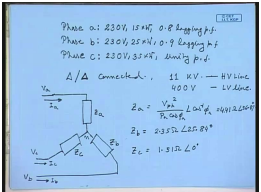The phase a load is 230 volt, 15 kilowatt at 0.8 lagging power factor, phase b load is 230 volt, 25 kilowatt with 0.9 lagging pf and phase c of a 3 phase transformer is supposed to supply this kind of loads 230 volt, 35 kilowatt at unity power factor. Now, find out let us say, the transformer is delta delta connected with no neural available. And the high voltage line side is 11 k V and the low voltage line 400 volts try to find out the voltage across each load phase.

Now, from the given load data these loads are star connected though, but the neutral of the load is isolated, since the transformer is delta connected the neutral is floating. So, the phase a load the load connection is somewhat like this is phase a load z a ,this is z b ,this is z c, this is the isolated neutral n, this is voltage V a , and this is the line current I a. similarly, this is the phase voltage V c, this is the line current I c, this is the phase voltage V b and this is the line current I b.

Now, from the given data, what is z a? z a equal to V phase square divided by p a cos phi a angle of cos inverse phi a and this comes to 4.41 ohm angle 36.87 degrees. Similarly z b equal to 2.35 ohm angle of 25.84 degrees, phase z c equal to 1.51 ohm angle of zero degrees. Now, this is a delta delta connected transformer this input side is connected to a balance 11k V source, and hence the low voltage side also you have balance 400 volts supply. Let us assume ideal transformer therefore, it will be convenient to replace the star connected load by an equivalent delta connected load.

(Refer Slide Time: 36:03)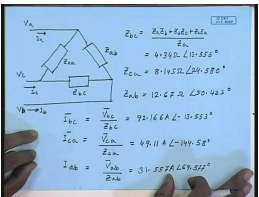The equivalent delta connected load will be connected like this, this is again V a this is the line current I and this is V c line current I c and this is V b line current I b, this is let us call it the impedance Z a b between a and b this is Z b c between b and c this is Z c a between c and a.

Now, from the star delta equivalence formula we can write z b c equal to z a z b plus z b Z c plus Z c Z a by Z a this comes to be equal to from the given data 4.34 ohm angle 13.553 degree. In the similar manner ,we can find out Z c a to be equal to 8.145 ohm angle 24.58 degrees , and Z a b comes out to be 12.67 ohm angle 50.423 degrees. Now, that we have the impedances it is easy to find out the currents let us say, I b c equal to V b c by Z b this comes to equal to 92.16 ampere at an angle of minus 13.53 degrees. Similarly, I c a will be V c a by Z c a equal to 49.1 ampere angle of minus 144.58 degrees, and I a b equal to V a b by Za b equal to 31.57 ampere angle 69.7 degrees. Now that we know the currents flowing through the equivalent delta connected loads.

(Refer Slide Time: 40:32)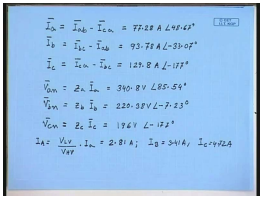We can proceed to find out the line current I a, I b and I c. I a is given by I a b minus I c a this comes to be 77.28 amperes angle point 6 7 degrees, I b come to I b c point I a b equal to 93.78 ampere angle minus 33.07 degrees, and I c line current equal to I c a minus I b c equal to one 29.8 ampere angle minus 177 degrees. Once we know the actual line currents it is now easy to find out the voltage across the different load phases. So, V a n will be equal to Z a into I a which comes out to be 340.8 volts angle 85.54 degrees. This V b n equal to Z b I b comes out to be 220.38 volt angle minus 7.23 degrees and V c n equal to Z c I c equal to 196 volt angle minus 177 degrees.

So, it is seen that the low voltages are highly unbalanced with minimum phase voltage magnitude of 196 volts and the maximum phase voltage magnitude of 341volts. So, this is the effect of unbalanced load when the load neutral is isolated. If we look at the h V side line currents in the particularly case that is I a, line current L V side of the transformer is delta connected h V side is also delta connected.

Therefore, I a equal to V L V by V HV into I a this comes to 2.81 amperes similarly, the line current I b comes to 3.41 amperes, and line current I c comes to 4.72. Both the load phase voltage and the input line currents are unbalanced, if the delta delta connected windings supplies a unbalanced star connected load.

(Refer Slide Time: 45:48)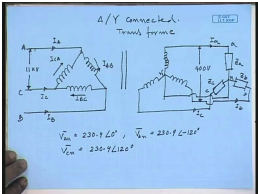Now, let us see what will happen if the transformer was delta star connected with the load neutral connected to the delta star connected transformer. Same set of loads, but now, the transformer is delta star connected. So, this is the arrangement. So, here there are applied line voltages 11 k V balanced line voltage is at the line currents I A, I C and I B. These are the lines A, C and B, this current winding current is I AB, this winding current is I BC and this winding current is I CA. The low voltage side is now star connected, and it supplies the same set of unbalanced load, but now the neutral of the load is connected to the neural of the low voltage winding.

This neutral is now connected to the load neutral this is Z a, this is Z b and this is Z c. This is the line current I a, this is the line current I b, and this is the line current I c. And the voltage between two lines here 400 volt. Now, since the load neutral is now connected to supply neutral the load phasors can operate independently and load phasors will be perfectly balanced that is V a n. It will be equal to 230.9angle 0, let us say then v b n would be equal to 230.9 angle minus 120, and V c n will be equal to 230.9 angle 130 degree.

(Refer Slide Time: 49:17)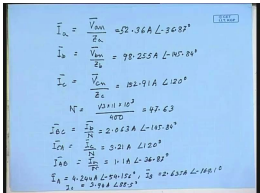It is easy to find out the line current in this case, the line currents I a will be V a n by Z a this comes to 52.36 ampere angle minus 36.87 degrees, I b equal to V b n by Z b equal to 98.25 amperes angle minus 145.84 degrees, I c equal to V c n by Z c equal to 152.91 amperes angle of 120 degrees. The transformer turns ratio now N equal to root 3 into 11 into 10 to the power 3 divided by 400 equal to 47.63. Therefore, I BC equal to I b by N equal to 2.063 amperes angle minus 145.84 degrees, I CA equal to I c by N equal to 3.21amperes angle of 120 degree, and I AB equal to I a bar by N equal to 1.1 ampere angle of minus 36.87 degrees. From this I can find out the high voltage side line currents IA this comes to 4.24 amperes angle of minus 54.156 degrees, I B comes out to be 2.635 amperes angle of minus point 169.1 degrees and I c comes out to be 3.94 amperes angle of 885 degrees.

So, we see that with neutral connection the load phase voltages are perfectly balanced; however, the high voltage side line currents are still unbalanced. So, that is the effect of unbalanced loading now. So, we have seen that where a star or delta connected winding should be used and it is why for almost all distribution transformers the second low voltage side is star connected with a neutral made available because the distribution transformers are expected to supply single mixed three phase and single phase load.

Now, one other point should be kept in mind, while operating three phase transformers in parallel. Since the parallel transformer line to neutral voltage should be same in magnitude and in phase. It is possible to connect 2, 3 phase transformers in parallel, only when in general they belong to the same vector group, because the transformers belonging to same vector group have line to neutral voltages, which are in phase, in addition the other conditions for parallel in 2 transformer namely, almost equal no load voltage, and the per unit series impedance of the transformer being equal should also be observed for paralleled 3 phase transformers.

Thank you.

Offer running on EduRev: Apply code STAYHOME200 to get INR 200 off on our premium plan EduRev Infinity!

61 docs|20 tests

,

,

,

,

,

,

,

,

,

,

,

,

,

,

,

,

,

,

,

,

,

;